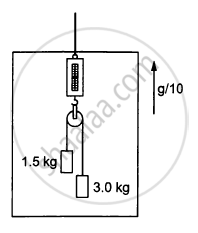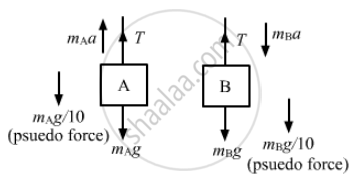# Find the Reading of the Spring Balance Shown in Figure (5−E6). the Elevator is Going up with an Acceleration G/10, the Pulley and the String Are Light and the Pulley is Smooth. - Physics

Sum

Find the reading of the spring balance shown in the following figure. The elevator is going up with an acceleration g/10, the pulley and the string are light and the pulley is smooth.#### Solution

Let the left and right blocks be A and B, respectively.
And let the acceleration of the 3 kg mass relative to the elevator be 'a' in the downward direction.From the free-body diagram,
$m_A a = T - m_A g - \frac{m_A g}{10} . . . \left( 1 \right)$
$m_B a = m_B g + \frac{m_B g}{10} - T . . . \left( 2 \right)$
Adding both the equations, we get:
$a\left( m_A + m_B \right) = \left( m_B - m_A \right)g + \left( m_B - m_A \right)\frac{g}{10}$
Putting value of the masses,we get:
$9a = \frac{33g}{10}$
$\Rightarrow \frac{a}{g} = \frac{11}{30} . . . \left( 3 \right)$
Now, using equation (1), we get:
$T = m_A \left( a + g + \frac{g}{10} \right)$
The reading of the spring balance =$\frac{2T}{g} = \frac{2}{g} m_A \left( a + g + \frac{g}{10} \right)$
$\Rightarrow 2 \times 1 . 5\left( \frac{a}{g} + 1 + \frac{1}{10} \right) = 3\left( \frac{11}{30} + 1 + \frac{1}{10} \right)$
= 4 . 4 kg

Concept: Newton’s Second Law of Motion
Is there an error in this question or solution?

#### APPEARS IN

HC Verma Class 11, Class 12 Concepts of Physics Vol. 1
Chapter 5 Newton's Laws of Motion
Q 16 | Page 80